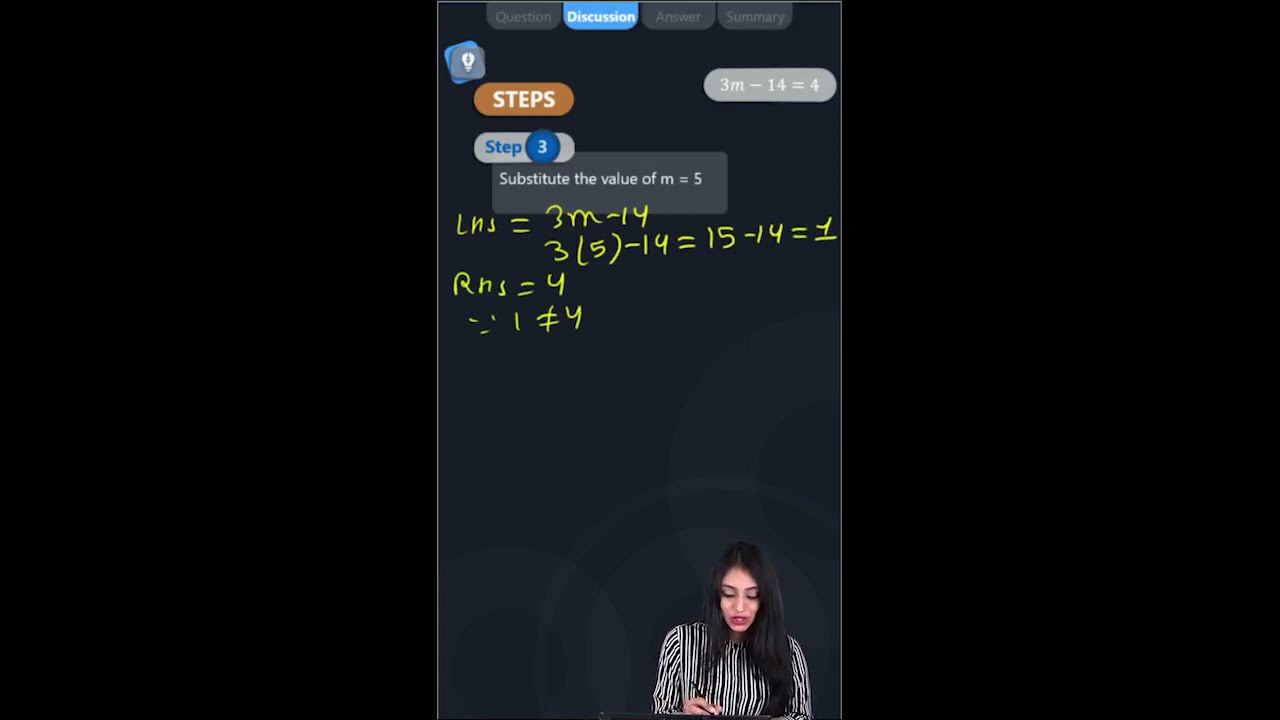Solve the equation by trial and error method: 3m – 14 = 4Given equation: 3m – 14 = 4

LHS = 3m – 14

By substituting the value of m = 3

Then,

LHS = 3m – 14

LHS = (3 × 3) – 14

LHS = 9 – 14

LHS = – 5

By comparing LHS and RHS

-5 ≠ 4

LHS ≠ RHS

Hence, the value of m = 3 is not a solution to the given equation.

Let, m = 4

LHS = 3m – 14

LHS = (3 × 4) – 14

LHS = 12 – 14

LHS = – 2

By comparing LHS and RHS

-2 ≠ 4

LHS ≠ RHS

Hence, the value of m = 4 is not a solution to the given equation.

Let, m = 5

LHS = 3m – 14

LHS = (3 × 5) – 14

LHS = 15 – 14

LHS = 1

By comparing LHS and RHS

1 ≠ 4

LHS ≠ RHS

Hence, the value of m = 5 is not a solution to the given equation.

Let, m = 6

LHS = 3m – 14

LHS = (3 × 6) – 14

LHS = 18 – 14

LHS = 4

By comparing LHS and RHS

4 = 4

LHS = RHS

Hence, the value of m = 6 is a solution to the given equation.(0)(0)